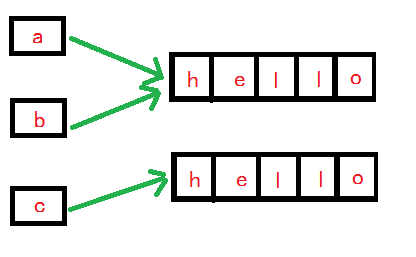# 深入理解 equals() 方法以及与 == 的区别

用知识改变命运，让我们的家人过上更好的生活

equals() 方法是Object 的一部分，因此所有的引用变量都可以调用equals() 方法来判断是否与其它的引用变量相等。使用这个方法，判断两个对象是否相等和 == 判断一样，只有引用变量指向同一个对象时才会返回true。因此通常情况下会重写equals 方法 。

• 自反性。对任意 x，x.equals(x) 一定返回 true。
• 对称性。对任意 x 和 y ，如果 y.equals(x) 返回 true，则 x.equals(y) 也返回true。
• 传递性。对任意 x、y、z，如果有 x.equals(y) 返回 true，y.equals(z) 返回true，则 x.equals(z) 也返回 true。
• 一致性。对任意 x 和 y，如果对象中用于等价比较的信息没有改变，那么无论调用 x.equals(y) 多少次，返回的结果应该保持一致，要么一直是 true，要么一直 是 false。
• 非空性。对于任何不是 null 的 x，x.equals(null) 一定返回 false。

equals() 方法和 == 的区别

• 如果两个变量是基本类型的变量，且都是数值类型，只要两个变量的数值相等，则返回 true

• 对于两个引用类型变量，== 比较的是变量(栈)内存中存放的对象的(堆)内存地址，用来判断两个对象的地址是否相同，只有它们指向同一个对象时，才会返回 true。

• == 比较的时候不一定要求数据类型一定相同，比如：int a = 100 ， long b= 100L 与 double c=100.0都是相同的，因为他们都指向地址为100的堆。

• ==不可用于比较类型上面没有父子关系的两个对象

• 默认的 Object.equals() 只是比较对象的地址。

String 的equals()方法判断两个字符串是否相等，只要两个字符串所包含的内容一样，则他们俩相等，返回true。• a == b , 返回true
• a== c，返回false
• a.equals( c），返回true
public class Test1 {
public static void main(String[] args) {
String s1 = new String("hello");
String s2 = new String("hello");
System.out.println("s1与s2是否相等: " + (s1 == s2)); // false
System.out.println("s1与s2是否相等: " + (s1.equals(s2))); // true

System.out.println("***********************************");
int a = 100;
long b = 100L;
char c = 'd';
System.out.println("a和b是否相等: " + (a == b)); // true
System.out.println("b和c是否相等: " + (b == c)); // true

System.out.println("***********************************");
Object o1 = new Object();
Object o2 = new Object();
System.out.println(o1 == o2); // false
System.out.println(o1.equals(o2)); // false
System.out.println(o1.toString().equals(o2.toString())); // false
}
}



s1与s2是否相等: false
s1与s2是否相等: true
***********************************
a和b是否相等: true
b和c是否相等: true
***********************************
false
false
false

public class Test2 {
public static void main(String[] args) {
String s1 = "springboot";
String s2 = "spring";
String s3 = "boot";
// 常量与常量的拼接结果存放在常量池
String s4 = "spring" + "boot";
// 拼接的时候，只要其中有一个是变量，结果就在堆中
String s5 = "spring" + s3;
String s6 = s2 + s3;
// new String()创建的字符串保存在堆内存
String s7 = new String("springboot");
// 如果拼接的结果调用intern()方法，返回值就在常量池中
String s8 = s6.intern();

System.out.println(s1 == s4); // true
System.out.println(s1 == s5); // false
System.out.println(s1 == s6); // false
System.out.println(s1 == s7); // false
System.out.println(s1 == s8); // true
}
}


true
false
false
false
true


11-071万+12-1339
09-26943
06-27470
03-201988
10-237930
08-25216
03-02284
03-2858
04-2915
04-2199
03-28542
05-2057
07-1963
09-072万+
05-11259
04-0957
11-248
01-17336